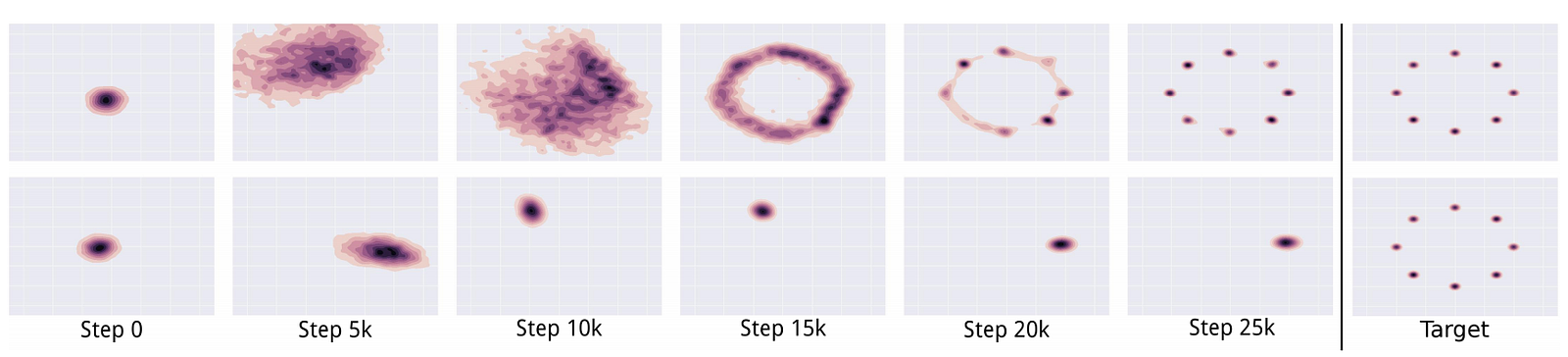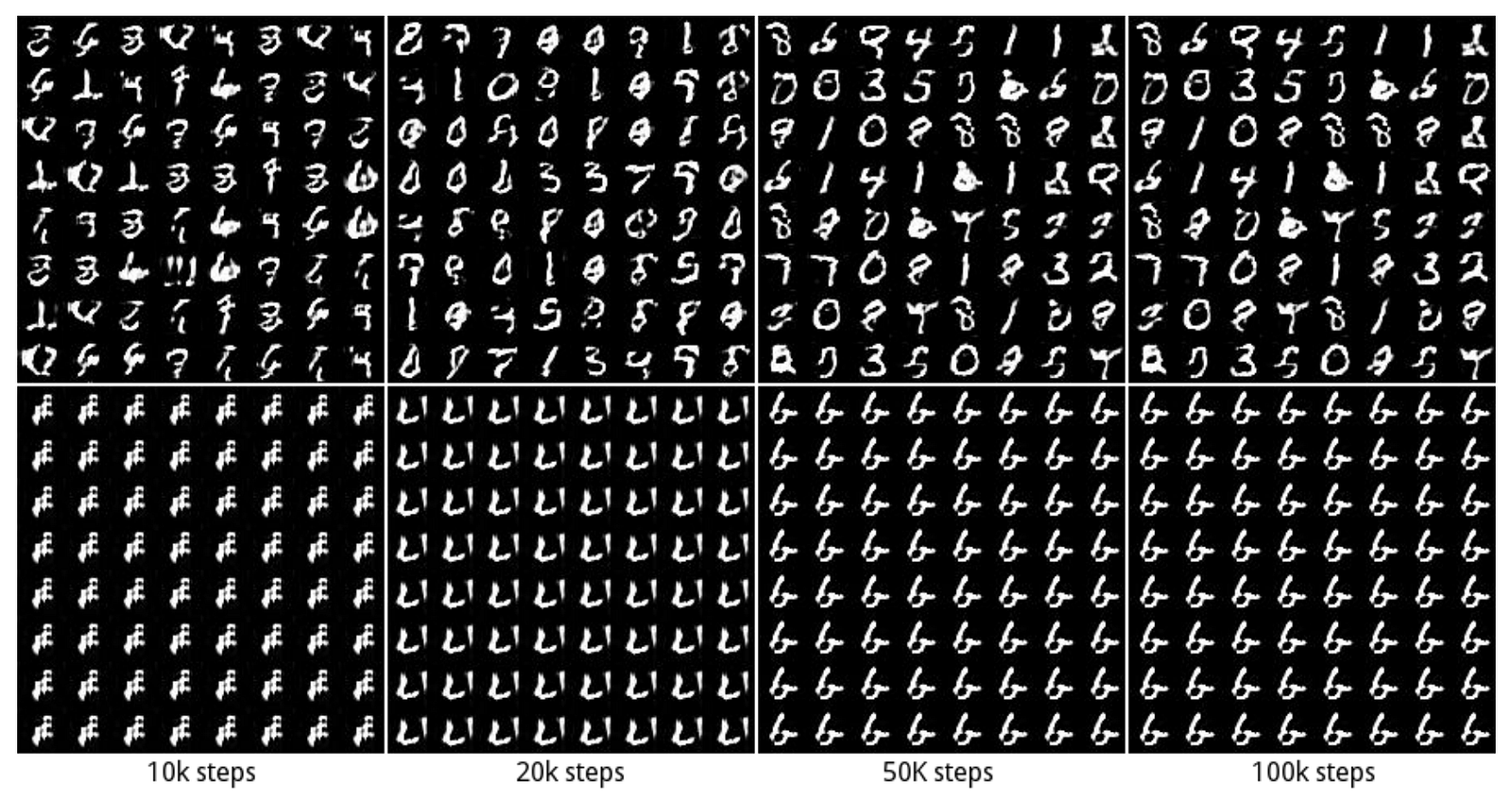# GAN — Unrolled GAN (how to reduce mode collapse)Photo by Ethan Hu

Intuition: In any game, you look ahead for the next few moves of your opponent and prepare your next move accordingly. In Unrolled GAN, we give an opportunity for the generator to unroll k steps on how the discriminator may optimize itself. Then we update the generator using backpropagation with the cost calculated in the final k step. The lookahead discourages the generator to exploit local optimal that easily counteract by the discriminator. Otherwise, the model will oscillate and even become unstable. Unrolled GAN lowers the chance that the generator is overfitted for a specific discriminator. This lessens mode collapse and improves stability.

This article is part of the series on GAN. Since mode collapse is common, we spend some time to explore Unrolled GAN to see how mode collapse may be addressed.

### Discriminator training

In GAN, we compute the cost function and use backpropagation to fit the model parameters of the discriminator D and the generator G.

We redraw the diagram below to emphasize the model parameters θ. The red arrows show how we backpropagate the cost function f to fit the model parameters.

Here are the cost function and the gradient descent. (we use a simple gradient descent for the purpose of the illustration)

In the diagram below, we add the SGD (gradient descent formula) to explicitly define how the discriminator parameters are calculated.

In Unrolled GAN, we train the discriminator exactly the same way as GAN.

### Generator training

Unrolled GAN plays k steps to learn how the discriminator may optimize itself for the specific generator. In general, we use 5 to 10 unrolled steps which demonstrates pretty good model performance. The diagram below unrolls the process 3 times.

The cost function is based on the latest discriminator’s model parameters while the generator’s model parameters remain the same.

At each step, we apply the gradient descent to optimize a new model for the discriminator.

But as mentioned before, we only use the first step to update the discriminator. The unrolling is used by the generator to predict moves but not used in the discriminator optimization. Otherwise, we may overfit the discriminator for a specific generator.

For the generator, we backpropagate the gradient throughout all k steps. This is very similar to how LSTM is unrolled and how gradients are backpropagated. Since we have k unrolled steps, the generator also accumulates the parameter changes k times (one for each step) as shown above.

To summarize, the Unrolled GAN uses the cost function calculated in the last step to perform the backpropagation for the generator while the discriminator uses the first step only.

### Coding

The implementation of Unrolled GAN can be found from here. Actually, it is pretty simple. The core logic for unroll k step is simply:

`for i in range(params['unrolling_steps'] - 1):    cur_update_dict = graph_replace(update_dict, cur_update_dict)    unrolled_loss = graph_replace(loss, cur_update_dict)`

which the graph_replace loads the discriminator with the latest discriminator model from the last step. Here is the core logic in building the computation graph in TensorFlow.

`with slim.arg_scope([slim.fully_connected],        weights_initializer=tf.orthogonal_initializer(gain=1.4)):    samples = generator(noise, output_dim=params['x_dim'])    real_score = discriminator(data)    fake_score = discriminator(samples, reuse=True)loss = tf.reduce_mean(          tf.nn.sigmoid_cross_entropy_with_logits(logits=real_score,              labels=tf.ones_like(real_score)) +          tf.nn.sigmoid_cross_entropy_with_logits(logits=fake_score,              labels=tf.zeros_like(fake_score)))...`
`updates = d_opt.get_updates(disc_vars, [], loss)d_train_op = tf.group(*updates, name="d_train_op")...`
`# Get dictionary mapping from variables to their update value# after one optimization stepupdate_dict = extract_update_dict(updates)cur_update_dict = update_dictfor i in range(params['unrolling_steps'] - 1):    cur_update_dict = graph_replace(update_dict, cur_update_dict)    unrolled_loss = graph_replace(loss, cur_update_dict)`
`...g_train_op = g_train_opt.minimize(-unrolled_loss, var_list=gen_vars)`
`...f, _, _ = sess.run([[loss, unrolled_loss], g_train_op, d_train_op])`

### Experiments

In the experiment below, we start with a toy dataset contains a mixture of 8 Gaussian distributions. Provided with a less complex generator, the GAN in the second row manages to generate good data quality but fail to achieve diversity. The mode collapses. Applying Unrolled GAN, it discovers all 8 modes with high quality (the first row).Source

RNN generator is particular vulnerable to mode collapse. The Unrolled GAN (the first row) manages to discover all 10 modes while a regular GAN model collapses (the second row).Source

### Further readings

If you want to learn more in improving GANs:

A full listing of all articles in this series:

### Reference

Unrolled GAN paper

Code implementation in TensorFlow